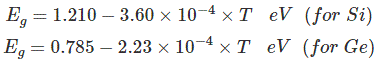# What is Semiconductor?

### Semiconductor

The semiconductor materials can be classified by the energy gap between their valence band and the conduction band. The valence band is the band consisting of the valence electron, and the conduction band remains empty. Conduction takes place when an electron jumps from valence band to conduction band and the gap between these two bands is forbidden energy gap. Wider the gap between the valence and conduction bands, higher the energy it requires for shifting an electron from valence band to the conduction band. In the case of conductors, this energy gap is absent or in other words conduction band, and valence band overlaps each other. Thus, electron requires minimum energy to jump from valence band. The typical examples of conductors are Silver, Copper, and Aluminium.

Also you can read: What is SCR

In insulators, this gap is vast. Therefore, it requires a significant amount of energy to shift an electron from valence to conduction band. Thus, insulators are poor conductors of electricity. Mica and Ceramic are the well-known examples of insulation material. Semiconductors, on the other hand, have an energy gap which is in between that of conductors and insulators. This gap is typically more or less 1 eV, and thus, one electron requires energy more than conductors but less than insulators for shifting valence band to conduction band. At low temperature there are very less number of electrons in conduction band in a semiconductor crystal but when the temperature is increased more and more electrons get sufficient energy to migrate from valence band to conduction band. Because of that, they don’t conduct electricity at low temperature but as the temperature increases the conductivity increases. The most typical examples of the semiconductors are silicon and germanium.

### Definition of Semiconductor

Thus, the definition of semiconductor can be as follows.

The materials that are neither conductor nor insulator with energy gap of about 1 eV (electron volt) are called semiconductors.

Most common type of materials that are commercially used as semiconductors are germanium (Ge) and silicon (Si) because of their property to withstand high temperature. That means there will be no significant change in energy gap with changing temperature. The relation between energy

gap and absolute temperature for Si and Ge are given as,Where, T = absolute temperature in oK
Assuming room temperature to be 300oK,### Operation

At room temperature resistivity of semiconductor is in between insulators and conductors. Semiconductors show negative temperature coefficient of resistivity that means its resistance decreases with increase in temperature. Both Si and Ge are elements of IV group i.e. both elements have 4 valence electrons. Both form the covalent bond with the neighboring atom. At absolute zero temperature both behave as insulator i.e. the valence band is full while conduction band is empty but as the temperature is raised more and more covalent bonds break and electrons are set free and jump to the conduction band.In the above energy band diagrams of a semiconductor. CB is the conduction band and VB is the valence band. At 0oK, the VB is full with all the valence electrons.